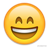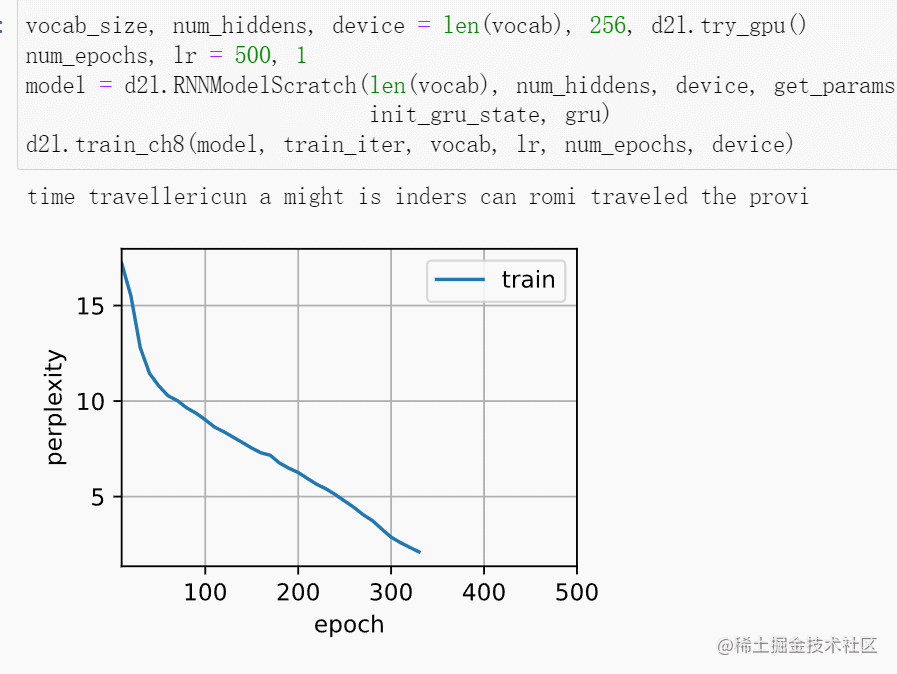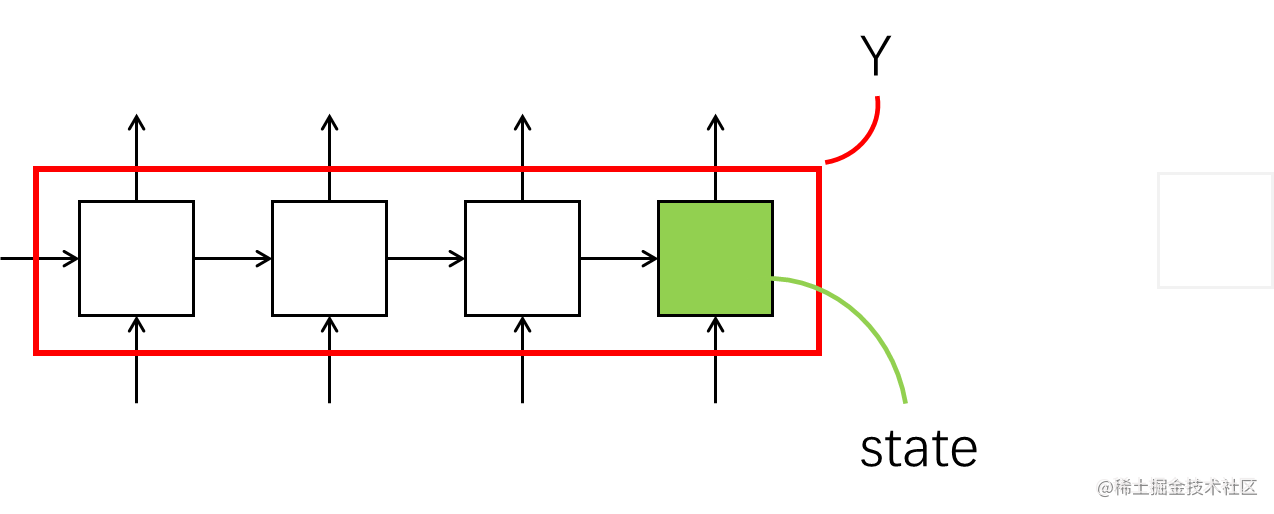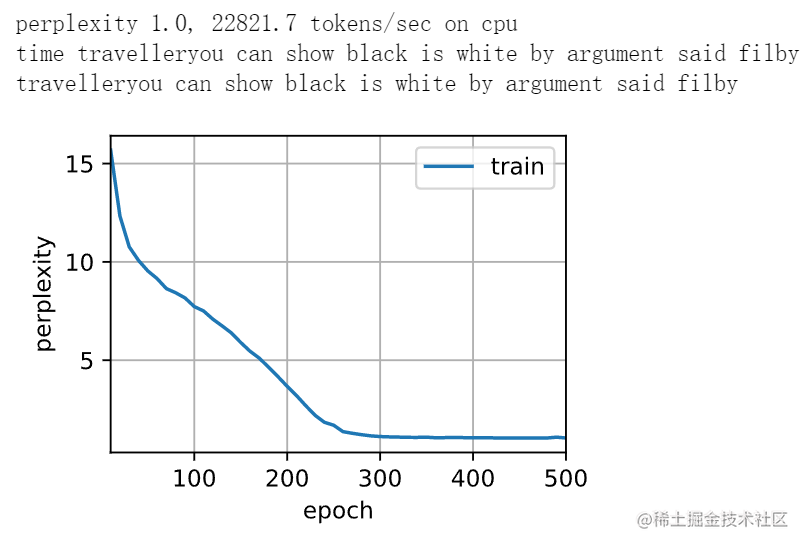# 用pytorch寫個 GRU 門控迴圈單元

## theme: awesome-green

1. 本文使用jupyter notebook寫的程式碼，和pycharmh有一點不一樣。比如x可以直接輸出變數，但是在pycharm中需要使用print(x)才可以。

# 自己寫

py import torch from torch import nn from d2l import torch as d2l

batch_size, num_steps = 32, 35 train_iter, vocab = d2l.load_data_time_machine(batch_size, num_steps) 

• 呼叫其他的包。

• 設定batch-size批量大小和時間步的長度num_step。這裡需要注意時間步的長度是你一個要處理的序列的時間步有多少個。

• 使用之前我們實現過的載入時光機器資料集。獲得資料集的迭代器和詞彙表的長度，這裡為了方便，使用的是char進行分割，也就是說詞彙表是a\~z以及空格和\<unk>。

py def get_params(vocab_size, num_hiddens, device): num_inputs = num_outputs = vocab_size

def normal(shape):

def three():
return (normal((num_inputs, num_hiddens)),
normal((num_hiddens, num_hiddens)),
torch.zeros(num_hiddens, device=device))

W_xz, W_hz, b_z = three()  # 更新門引數
W_xr, W_hr, b_r = three()  # 重置門引數
W_xh, W_hh, b_h = three()  # 候選隱藏狀態引數

W_hq = normal((num_hiddens, num_outputs))
b_q = torch.zeros(num_outputs, device=device)

params = [W_xz, W_hz, b_z, W_xr, W_hr, b_r, W_xh, W_hh, b_h, W_hq, b_q]
for param in params:
return params


 看程式碼之前記得回顧一下GRU的計算公式： \begin{aligned} &重置門：&\mathbf{R}t = \sigma(\mathbf{X}_t \mathbf{W}{xr} + \mathbf{H}{t-1} \mathbf{W}{hr} + \mathbf{b}r),\ &更新門：&\mathbf{Z}_t = \sigma(\mathbf{X}_t \mathbf{W}{xz} + \mathbf{H}{t-1} \mathbf{W}{hz} + \mathbf{b}z), \ &候選隱藏狀態：&\tilde{\mathbf{H}}_t = \tanh(\mathbf{X}_t \mathbf{W}{xh} + \left(\mathbf{R}t \odot \mathbf{H}{t-1}\right) \mathbf{W}{hh} + \mathbf{b}_h) \ &隱藏狀態：&\mathbf{H}_t = \mathbf{Z}_t \odot \mathbf{H}{t-1} + (1 - \mathbf{Z}_t) \odot \tilde{\mathbf{H}}_t \end{aligned}

• num_hiddens 定義隱藏單元的數量，

• normal函式用於從標準差為 0.01 的高斯分佈中隨機生成權重。

• three函式用於給更新門、重置門、候選隱藏狀態初始化權重和偏執，一次更新仨就叫three了。• 後邊三個w,w,b = three()分別對應更新門、重置門、候選隱藏狀態的初始化。

• w_hqb_q是初始化隱藏層到輸出層的權重和偏執。

• params將引數整理到一起，為其附加梯度。

py def init_gru_state(batch_size, num_hiddens, device): return (torch.zeros((batch_size, num_hiddens), device=device), ) 這個是隱狀態初始化函式。將其初始化為0張量。

py def gru(inputs, state, params): W_xz, W_hz, b_z, W_xr, W_hr, b_r, W_xh, W_hh, b_h, W_hq, b_q = params H, = state outputs = [] for X in inputs: Z = torch.sigmoid((X @ W_xz) + (H @ W_hz) + b_z) R = torch.sigmoid((X @ W_xr) + (H @ W_hr) + b_r) H_tilda = torch.tanh((X @ W_xh) + ((R * H) @ W_hh) + b_h) H = Z * H + (1 - Z) * H_tilda Y = H @ W_hq + b_q outputs.append(Y) return torch.cat(outputs, dim=0), (H,)

• 開始是用params設定gru的引數。

• H獲取初始的隱藏狀態

• for迴圈就是對其進行計算。對照公式一目瞭然的東西。

py vocab_size, num_hiddens, device = len(vocab), 256, d2l.try_gpu() num_epochs, lr = 500, 1 model = d2l.RNNModelScratch(len(vocab), num_hiddens, device, get_params, init_gru_state, gru) d2l.train_ch8(model, train_iter, vocab, lr, num_epochs, device) 這段程式碼是對我們手寫GRU的訓練和預測測試，會輸出預測結果和訓練過程的視覺化。給你們看個好玩的，仔細看輸出的句子和影象。隨著訓練句子會一直改變，困惑度也一直下降。在CPU上會訓練很久，所以要等好長一會兒讓他跑完500個epoch。# 呼叫人家的

py import torch from torch import nn from d2l import torch as d2l from torch.nn import functional as F 依舊是熟悉的配方熟悉的導包操作。

py batch_size, num_steps = 32, 35 train_iter, vocab = d2l.load_data_time_machine(batch_size, num_steps) 這裡和之前沒區別，設定一些超引數並載入資料集。

• 設定batch-size批量大小和時間步的長度num_step。這裡需要注意時間步的長度是你一個要處理的序列的時間步有多少個。

• 使用之前我們實現過的載入時光機器資料集。獲得資料集的迭代器和詞彙表的長度，這裡為了方便，使用的是char進行分割，也就是說詞彙表是a\~z以及空格和\<unk>。

py class GRUModel(nn.Module): def init(self, gru_layer, vocab_size, kwargs): super(GRUModel, self).init(kwargs) self.gru = gru_layer self.vocab_size = vocab_size self.num_hiddens = self.gru.hidden_size if not self.gru.bidirectional: self.num_directions = 1 self.linear = nn.Linear(self.num_hiddens, self.vocab_size) else: self.num_directions = 2 self.linear = nn.Linear(self.num_hiddens * 2, self.vocab_size)

def forward(self, inputs, state):
X = F.one_hot(inputs.T.long(), self.vocab_size)
X = X.to(torch.float32)
Y, state = self.gru(X, state)
output = self.linear(Y.reshape((-1, Y.shape[-1])))
return output, state

def begin_state(self, device, batch_size=1):
return  torch.zeros((self.num_directions * self.gru.num_layers,
batch_size, self.num_hiddens),
device=device)


 RNN、GRU、LSTM一脈相承，用的類的都差不多，這個是適用於RNN和GRU的，但是不適用於LSTM。LSTM可以看我之後的文章，或者看前邊的[簡潔實現RNN迴圈神經網路 ](https://juejin.cn/post/7071225202205523975)實現的那個RNNModule類，那個類是涵蓋了RNN、GRU、LSTM的通用模型。 -init初始化這個類，這個類是繼承了nn.Module的。

- self.gru設定計算層是GRU層，這裡是需要引數的，你在下一段程式碼中會傳入nn.GRU。

- self.vocab_size設定字典的大小，這裡大小是28，因為我們使用的是字母進行分詞，所以其中只有a~z26個字母外加 和<unk>（空格和unknown）。
- self.num_hiddens設定隱藏層的大小。普通的RNN是隱藏層，在這裡是帶隱狀態的隱藏層。不是說有隱狀態之後就沒隱藏層了。
- if-else語句是設定GRU是單雙向的。

• forward定義前向傳播網路。

這裡不用我們自己來實現計算過程了，nn.GRU會直接給我們計算。但是我們依舊需要對資料進行一下才操作。

• 首先是將輸入轉化為對應的one-hot向量，這裡F看前邊導包部分，是使用nn.functional

• torch.float32再將其型別轉化為float。
• Ystate是計算隱狀態的，注意 在這裡Y不是 輸出。這裡Y是輸出全部的隱狀態，state是輸出最後一個時間步的隱狀態。- output是用於儲存輸出的。 - begin_state是進行初始化。 這裡初始化和RNN初始化一樣，都是初始化為一個零張量。之後可以留意一下LSTM，LSTM是返回一個元組，元組中有兩個張量。

py vocab_size, num_hiddens, device = len(vocab), 256, d2l.try_gpu() num_epochs, lr = 100, 1 num_inputs = vocab_size gru_layer = nn.GRU(num_inputs, num_hiddens) model = GRUModel(gru_layer, len(vocab)) model = model.to(device) d2l.train_ch8(model, train_iter, vocab, lr, num_epochs, device)

• num_hiddens隱藏層向量的長度
• device在CPU還是GPU上執行
• num_epochs訓練的epoch數量
• lr學習率learning rate

GRU層直接使用nn.GRU